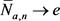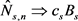# Gt/Gt/1队列模型稳态性能指标的研究Study on Steady-State Performance Measures of the Gt/Gt/1 Queue Model

• 全文下载: PDF(530KB)    PP.706-711   DOI: 10.12677/PM.2018.86095
• 下载量: 260  浏览量: 360

For the Gt/Gt/1 single-server queue model with time-varying arrival rate, we suppose that the waiting space is infinite. By combining the knowledge of the Stochastic-Process Limit and the Convergence of Probability Measures, then the convergence limits of the steady-state performance measures in the queue model are obtained on the basis of a given arrival rate function

1. 引言

2. Gt/Gt/1队列模型

$\Lambda \left(t\right)\equiv {\int }_{0}^{t}\lambda \left(s\right)\text{d}s$ ,

$\stackrel{¯}{\lambda }\equiv \underset{t\to \infty }{\mathrm{lim}}\frac{\Lambda \left(t\right)}{t}$ ,

$A\left(t\right)\equiv {N}_{a}\left(\Lambda \left(t\right)\right)={N}_{a}\left({\int }_{0}^{t}\lambda \left(s\right)\text{d}s\right)$ ,, ${\stackrel{^}{N}}_{a,n}⇒{c}_{a}{B}_{a}$ ,

${\stackrel{¯}{N}}_{s,n}\to e$ ,,

3. 主要结论及讨论

${\stackrel{¯}{\lambda }}_{n}\left(t\right)\equiv {\lambda }_{n}\left(nt\right)$ , ${\stackrel{¯}{\Lambda }}_{n}\left(t\right)\equiv \frac{{\Lambda }_{n}\left(nt\right)}{n}$ .

${\stackrel{˜}{\Lambda }}_{n,t}\left(u\right)\equiv \frac{{\Lambda }_{n}\left(nt+u\sqrt{n}\right)-{\Lambda }_{n}\left(nt\right)}{\sqrt{n}}$ , $t\ge 0$ , $n\ge 1$ .

${\stackrel{^}{\Lambda }}_{n}\left(t\right)\equiv \frac{{\Lambda }_{n}\left(nt\right)-n{\Lambda }_{f}\left(t\right)}{\sqrt{n}}$ ,

${A}_{n}\left(t\right)\equiv {N}_{a}\left({\Lambda }_{n}\left(t\right)\right)={N}_{a}\left({\int }_{0}^{t}{\lambda }_{n}\left(s\right)\text{d}s\right)$ ,

${\stackrel{¯}{A}}_{n}\left(t\right)\equiv \frac{{N}_{a}\left({\Lambda }_{n}\left(nt\right)\right)}{n}$ , ${\stackrel{^}{A}}_{n}\left(t\right)\equiv \frac{{A}_{n}\left(nt\right)-n{\Lambda }_{f}\left(t\right)}{\sqrt{n}}$ ,

${\stackrel{˜}{A}}_{n,t}\left(u\right)\equiv \frac{{A}_{n}\left(nt+u\sqrt{n}\right)-{A}_{n}\left(nt\right)}{\sqrt{n}}$ , $t\ge 0$ , $n\ge 1$ .

${\stackrel{˜}{A}}_{n,t}\left(u\right)\to {\lambda }_{f}\left(t\right)u$ .

${\stackrel{¯}{A}}_{n}\left(t\right)\equiv \frac{{N}_{a}\left({\Lambda }_{n}\left(nt\right)\right)}{n}\text{=}\frac{n{\stackrel{¯}{N}}_{a,n}\left(\frac{{\Lambda }_{n}\left(nt\right)}{n}\right)}{n}={\stackrel{¯}{N}}_{a,n}\left(\frac{{\Lambda }_{n}\left(nt\right)}{n}\right)={\stackrel{¯}{N}}_{a,n}\circ {\stackrel{¯}{\Lambda }}_{n}\to {\Lambda }_{f}$ ,

$\begin{array}{l}{\stackrel{^}{A}}_{n}\left(t\right)\equiv \frac{{A}_{n}\left(nt\right)-n{\Lambda }_{f}\left(t\right)}{\sqrt{n}}=\frac{{A}_{n}\left(nt\right)}{\sqrt{n}}-\sqrt{n}{\Lambda }_{f}\left(t\right)=\frac{{N}_{a}\left({\Lambda }_{n}\left(nt\right)\right)}{\sqrt{n}}-\sqrt{n}{\Lambda }_{f}\left(t\right)\\ \text{\hspace{0.17em}}\text{\hspace{0.17em}}\text{\hspace{0.17em}}\text{\hspace{0.17em}}\text{\hspace{0.17em}}\text{\hspace{0.17em}}\text{\hspace{0.17em}}\text{\hspace{0.17em}}=\frac{\sqrt{n}{\stackrel{^}{N}}_{a,n}\left(\frac{{\Lambda }_{n}\left(nt\right)}{n}\right)+{\Lambda }_{n}\left(nt\right)}{\sqrt{n}}-\sqrt{n}{\Lambda }_{f}\left(t\right)={\stackrel{^}{N}}_{a,n}\left(\frac{{\Lambda }_{n}\left(nt\right)}{n}\right)+\frac{{\Lambda }_{n}\left(nt\right)}{\sqrt{n}}-\sqrt{n}{\Lambda }_{f}\left(t\right)\\ \text{\hspace{0.17em}}\text{\hspace{0.17em}}\text{\hspace{0.17em}}\text{\hspace{0.17em}}\text{\hspace{0.17em}}\text{\hspace{0.17em}}\text{\hspace{0.17em}}\text{\hspace{0.17em}}={\stackrel{^}{N}}_{a,n}\left({\stackrel{¯}{\Lambda }}_{n}\left(t\right)\right)+\frac{{\Lambda }_{n}\left(nt\right)-n{\Lambda }_{f}\left(t\right)}{\sqrt{n}}={\stackrel{^}{N}}_{a,n}\circ {\stackrel{¯}{\Lambda }}_{n}\left(t\right)+{\stackrel{^}{\Lambda }}_{n}\left(t\right)\\ \text{\hspace{0.17em}}\text{\hspace{0.17em}}\text{\hspace{0.17em}}\text{\hspace{0.17em}}\text{\hspace{0.17em}}\text{\hspace{0.17em}}\text{\hspace{0.17em}}\text{\hspace{0.17em}}⇒{c}_{a}{B}_{a}\circ {\Lambda }_{f}+{\Lambda }_{d}\end{array}$

${\stackrel{^}{N}}_{a,n}⇒{c}_{a}{B}_{a}$ ，以及胎紧性，有： $\frac{{N}_{a}\left(nt+u\sqrt{n}\right)-{N}_{a}\left(nt\right)}{\sqrt{n}}⇒u$ ，进而得：

$\begin{array}{l}{\stackrel{˜}{A}}_{n,t}\left(u\right)\equiv \frac{{A}_{n}\left(nt+u\sqrt{n}\right)-{A}_{n}\left(nt\right)}{\sqrt{n}}\\ \text{\hspace{0.17em}}\text{\hspace{0.17em}}\text{\hspace{0.17em}}\text{\hspace{0.17em}}\text{\hspace{0.17em}}\text{\hspace{0.17em}}\text{\hspace{0.17em}}\text{\hspace{0.17em}}\text{\hspace{0.17em}}\text{\hspace{0.17em}}=\frac{{N}_{a}\left({\Lambda }_{n}\left(nt+u\sqrt{n}\right)\right)-{N}_{a}\left({\Lambda }_{n}\left(nt\right)\right)}{\sqrt{n}}\\ \text{\hspace{0.17em}}\text{\hspace{0.17em}}\text{\hspace{0.17em}}\text{\hspace{0.17em}}\text{\hspace{0.17em}}\text{\hspace{0.17em}}\text{\hspace{0.17em}}\text{\hspace{0.17em}}\text{\hspace{0.17em}}\text{\hspace{0.17em}}=\frac{{N}_{a}\left({\Lambda }_{n}\left(nt\right)+{\lambda }_{f}\left(t\right)u\sqrt{n}+ο\left(\sqrt{n}\right)\right)-{N}_{a}\left({\Lambda }_{n}\left(nt\right)\right)}{\sqrt{n}}\\ \text{\hspace{0.17em}}\text{\hspace{0.17em}}\text{\hspace{0.17em}}\text{\hspace{0.17em}}\text{\hspace{0.17em}}\text{\hspace{0.17em}}\text{\hspace{0.17em}}\text{\hspace{0.17em}}\text{\hspace{0.17em}}\text{\hspace{0.17em}}⇒{\lambda }_{f}\left(t\right)u\end{array}$

$t\ge 0$ 时， ${\stackrel{^}{Q}}_{n}\left(t\right)\equiv \frac{{Q}_{n}\left(nt\right)}{\sqrt{n}}$${\stackrel{^}{W}}_{n}\left(t\right)\equiv \frac{{W}_{n}\left(nt\right)}{\sqrt{n}}$

$R\left(t;a,b\right)$ 是漂移系数为a，扩散系数为b的反射布朗运动。

${\stackrel{^}{A}}_{n}\left(t\right)\equiv \frac{{N}_{a}\left(nt\right)-nt}{\sqrt{n}}$

${\stackrel{^}{S}}_{n}\left(t\right)\equiv \frac{{N}_{s}\left(nt/{\rho }_{n}\right)-nt}{\sqrt{n}}=\frac{{N}_{s}\left(nt/{\rho }_{n}\right)-nt/{\rho }_{n}}{\sqrt{n}}+\frac{\sqrt{n}}{\sqrt{n}-1}t$

$\left({\stackrel{^}{A}}_{n},{\stackrel{^}{S}}_{n}\right)⇒\left({B}_{a},{B}_{s}+e\right)$

${\stackrel{^}{Q}}_{n}⇒R\left(\cdot \right)\equiv R\left(\cdot ;-1,{c}_{a}^{2}+{c}_{s}^{2}\right)$ ，且 ${\stackrel{^}{Q}}_{n}⇒R\left({\Lambda }_{f}\left(\cdot \right)\right)$

${D}_{n}\left(t\right)$ 代表第n个系统中的离去过程，

$\begin{array}{c}{\stackrel{^}{W}}_{n}\left(t\right)\equiv \frac{{W}_{n}\left(nt\right)}{\sqrt{n}}=\mathrm{inf}\left\{u\ge 0:{D}_{n}\left(nt+u\sqrt{n}\right)-{D}_{n}\left(nt\right)\ge {Q}_{n}\left(nt\right)\right\}\\ =\mathrm{inf}\left\{u\ge 0:\left({D}_{n}\left(nt+u\sqrt{n}\right)-{D}_{n}\left(nt\right)\right)/\sqrt{n}\ge {Q}_{n}\left(nt\right)/\sqrt{n}\right\}\\ =\mathrm{inf}\left\{u\ge 0:{\stackrel{^}{D}}_{n,t}\left(u\right)\ge {\stackrel{^}{Q}}_{n}\left(t\right)\right\}\end{array}$ ,

${B}_{n}\left(t\right)$$\left[0,t\right]$ 时间内忙的服务台。

${D}_{n}\left(nt\right)={N}_{s}\left({\rho }_{n}^{-1}{B}_{n}\left({\Lambda }_{n}\left(nt\right)\right)\right)$ , $t\ge 0$

$\frac{{B}_{n}\left({\Lambda }_{n}\left(nt+u\sqrt{n}\right)\right)-{B}_{n}\left({\Lambda }_{n}\left(nt\right)\right)}{\sqrt{n}}\to u{\lambda }_{f}\left(t\right)$ ,

${\stackrel{¯}{N}}_{s,n}\to e$${\stackrel{^}{N}}_{s,n}⇒{c}_{s}{B}_{s}$${\stackrel{^}{D}}_{n,t}\left(u\right)\to u{\lambda }_{f}\left(t\right)$

${\stackrel{^}{Q}}_{n}⇒\stackrel{^}{Q}$ ，及 $\stackrel{^}{W}\left(t\right)\equiv \frac{\stackrel{^}{Q}\left(t\right)}{{\lambda }_{f}\left(t\right)}$$\stackrel{^}{Q}\left(t\right)\equiv R\left({\Lambda }_{f}\left(t\right);-1,{c}_{a}^{2}+{c}_{s}^{2}\right)$ ，得：

${D}^{2}$ 空间中，当 $n\to \infty$ 时，有 $\left({\stackrel{^}{Q}}_{n},{\stackrel{^}{W}}_{n}\right)⇒\left(\stackrel{^}{Q},\stackrel{^}{W}\right)$

4. 总结

  Ward, A.R. and Glynn, P.W. (2003) A Diffusion Approximation for a Markorvian Queue with Reneing. Queuing Systems, 43, 103-128.  Ward, A.R. and Glynn, P.W. (2005) A Diffusion Approximation for a Heavy-Traffic Limits for a GI/GI/1 Queue with Balking or Reneging. Queuing Systems, 50, 371-400.  Jelenkovic, P.R. (1999) Subexponential Loss Rates in GI/GI/1 Queue with Applications. Queuing Systems, 33, 91-123.  Whitt, W. (2004) Heavy-Traffic Limits for Loss Proportions in Single-Server Queues. Queuing Systems, 46, 507-536.  Liu, Y.N. and Whitt, W. (2014) Stabilizing Performance in Networks of Queues with Time-Varying Arrival Rates. Probability in the Engineering and Information Sciences, 28, 419-449. https://doi.org/10.1017/S0269964814000084  Whitt, W. (2016) Heavy-Traffic Limits for a Single-Server Queue Leading up to a Critical Point. Operations Research Letters, 44, 796-800. https://doi.org/10.1016/j.orl.2016.10.005  Ma, N. and Whitt, W. (2018) A Rare-Event Simulation Algorithm for Periodic Single-Server Queues. Informs Journal on Computing, 30, 71-89. https://doi.org/10.1287/ijoc.2017.0766  Dong, J. and Whitt, W. (2015) Using Birth-and-Death Processes to Estimate the Steady-State Distribution of A Periodic Queue. Naval Research Logistics, 62, 664-685. https://doi.org/10.1002/nav.21672  Baccelli, F., Boyer, P. and Hebuterne, G. (1984) Single-Server Queues with Impatient Customers. Journal of Applied Probability and Advances in Applied Probability, 16, 887-905.  Stanford, R.E. (1979) Renging Phenomena in Single Channel Queue. Mathematics of Operations Research, 4, 162-178. https://doi.org/10.1287/moor.4.2.162  Whitt, W. (2002) Stochastic-Process Limits. Springer, New York.  Billingsley, P. (1999) Convergence of Probability Measures. 2nd Edition. Wiley, New York. https://doi.org/10.1002/9780470316962